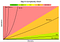# Understanding Big O Notation + Frequency Counter Pattern

## Time and Space complexityImages by Pixabay
1. What problem are we trying to solve?
2. Optimizing quadratic to linear function
3. Solving the problem with Frequency Counter Pattern
4. Comparing the two solutions using Big-O Complexity Chart
5. Conclusion

## O(1), constant

When an algorithm has O(1), the input is independent of time and space. Thus, time and space remain the same regardless of the input size.

## O(n), linear

When an algorithm has O(n), the input is dependent on time and space and increases linearly. Thus, time and space grow in direct proportion to the size of the input.

## O(n²), quadratic

When an algorithm has O(n²), the input is dependent on time and space and increases quadratically. Thus, time and space grow in direct proportion to the square of the size (n*n) of the input.

## O(2^n), exponential

When an algorithm has O(2^n), the algorithm time/space complexity doubles if there’s an addition to the input.

## O(logn), logarithms

I will use the binary search example to explain how the logarithm works. Binary search is a search algorithm used in finding the index of an element in an array. This is done by dividing the search/array into half each time we are looking for the target. With this, the time complexity is proportional to the logarithm of the input size.

# Given a function that takes two arrays as an argument, determine if the values are the same in both arrays.

In solving this problem, let’s first breakdown the question into smaller parts and make possible test cases.

• While the two params have the same size, I looped through the first array, and within the first array for loop block, I will loop through the second array. This is what is referred to as the “Nested loop”.
• The last thing I did was check if array1 does not include a value from array two at an index (which “j” in the code above). If that’s the case, then the two arrays are not the same, and therefore, I return false else, I return true.
• The array in stored in an object where each key (each array index) has a value (the number of times the key appears in the array). Using “for of” loop, I checked if the key already has a pair and increment the value or initialize to 1. This is done for both array1 and array2.
• The last I did was to use “for in” loop to loop through one of the objects, in this case frequencyCounter1. This way, I can check if a key in frequencyCounter1 can not be found in frequencyCounter2. If that’s the case, then that means the objects do not have the same key value pairs. If they have same values and keys, then I return true.Image by BigOCheatsSheet

Full-Stack Developer 👩‍💻|Content Management😊| Cooking👩‍🍳 | Sports🏃‍♀️

## More from Josephine Gyamera

Full-Stack Developer 👩‍💻|Content Management😊| Cooking👩‍🍳 | Sports🏃‍♀️